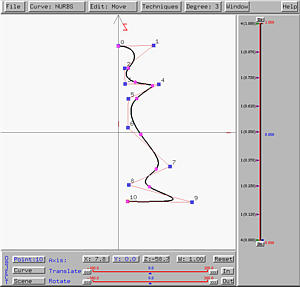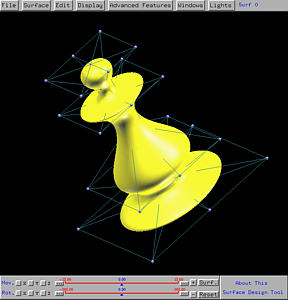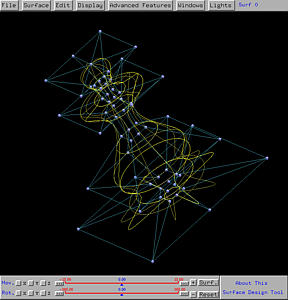# Surfaces of RevolutionA surface of revolution is generated by revolving a given curve about an axis. The given curve is a profile curve while the axis is the axis of revolution..

To design a surface of revolution, select Advanced Features followed by Cross Sectional Design. This will bring up the curve system. In the curve system, just design a profile curve based on the condition to be discussed below, and then select Techniques followed by Generate Surface of Revolution. The surface system will display a surface of revolution defined by the given profile curve.

Some special restrictions must be followed in order to design a surface of revolution under the curve system. First, the axis of revolution must be the z-axis. Second, the profile curve must be in the xz-plane. However, when brining up the curve system, only the xy-plane is shown. To overcome this problem, one can design a profile curve on the xy-plane and rotate the curve (not the scene) about the x-axis 90 degree (or -90 degree, depending on your need). In this way, the profile curve will be placed on the xz-plane. Note that to modify control points after this rotation, you must use the sliders.

Many commonly seen and useful surfaces are surfaces of revolution (e.g., spheres, cylinders, cones and tori). Here are a few examples.### Example 1: Sphere

Let us try the sphere first. A sphere is obtained by revolving a semi-circle about the axis of revolution. In the curve system, this semi-circle must be in the xz-plane and the axis of revolution must be the z-axis. The following semi-circle is a NURBS curve of degree 2. It has already been placed on the xz-plane so that its two endpoints are on the z-axis. Click here to download a copy of this file (sphere.dat) for your practice.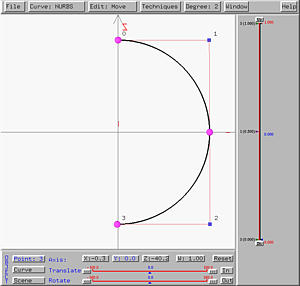The following shows the generated sphere, shown in rendered patch and wireframe. The top center and bottom center control points correspond to the two endpoints of the semi-circle. Note also the circular wireframe lines which are the loci of points on the semi-circle.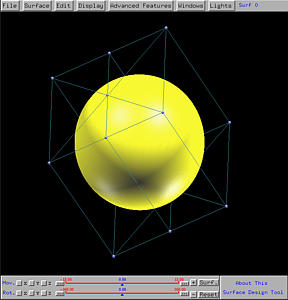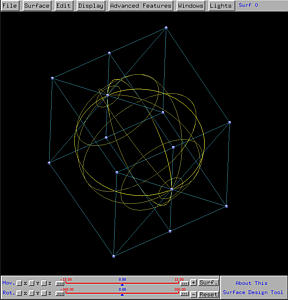### Example 2: Cone

A cone can also be generated as a surface of revolution. The profile curve is a line segment on a plane that contains the axis of revolution. The following figure shows a line segment that intersects the axis of revolution. Click here to download a copy of this file (cone.dat) for your practice.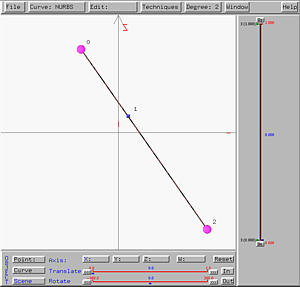The following shows the generated cone, shown in rendered patch and wireframe. Note that the intersection point of the profile curve and the axis of revolution becomes the vertex (or apex) of the cone. Note also that the profile line segment does not have to intersect the axis of revolution. In this case, the cone's vertex (or apex) will disappear. You can move the line segment away from the z-axis and generate a new surface of revolution to see the effect.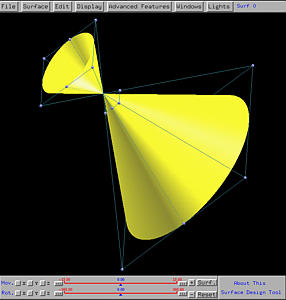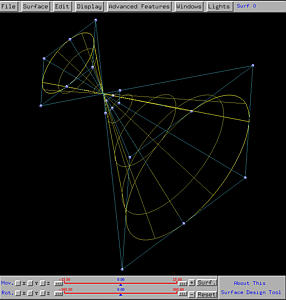### Example 3: Torus

The torus is another well-known surface of revolution and is generated by revolving a circle about an axis. The following figure shows a circle in the xz-plane. Click here to download a copy of this file (torus.dat) for your practice.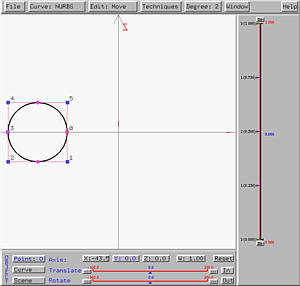The following shows the generated torus, shown in rendered patch and wireframe. The input circle does not intersect the axis of revolution. If you move the circle so that it is tangent or intersect the axis of revolution, the generate torus will not look like a donuts. For the tangent (resp., intersecting) case, the generate torus is referred to as a spindle torus with one (resp., two) singularities.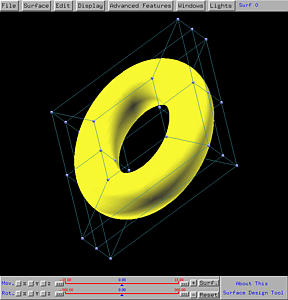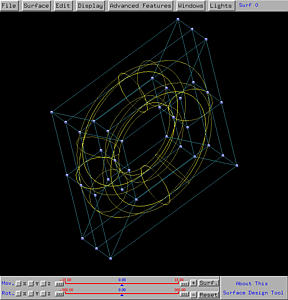### Example 4: Vase

Now, let us turn to some interesting surface design. Let us do a vase. First, design a profile curve, which looks like the profile of a vase, on the xy-plane and rotate it 90 degree to the xz-plane as shown below. This is a NURBS curve of degree 3. In general, degree three NURBS or even B-spline curves work well. Click here to download a copy of this file (vase.dat) for your practice.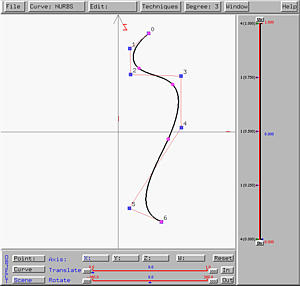The generate vase-like surface is shown below in both rendered patch and wireframe forms.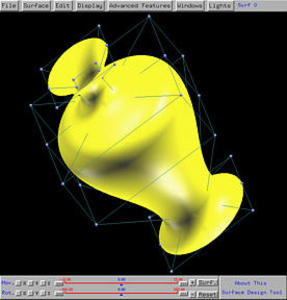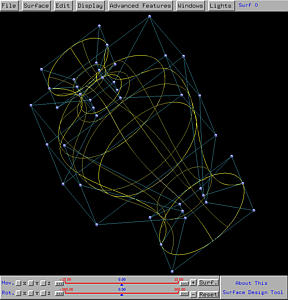### Example 5: Chessman